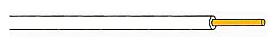﻿ Conductance Calculator ﻿# Conductance Calculator### Conductance Calculator By Power Formula (if Power and Current/Voltage are Known)Enter the Power and Voltage or Current to compute result.

 Power, P: W (Watts) mW (milliwatts) Voltage, V: V (volts) mV (millivolts) Current, I: A (amperes) mA (milliamperes) µA (microamperes)
0.00 S

This conductance calculator calculates the conductance of an electronic component based on the power that the component consumes and either the voltage which is dropped across it or the current going through it, according to the formulas, G= P/V2 (if power and voltage are known) or G=I2/P (if power and current are known).

To use this calculator, a user enters the power, P, and either the current, I, or the voltage, V, and the result will automatically be calculated and shown. The conductance result which is displayed above is in unit siemens (S).

### Conductance Calculator By Resistivity Formula (if resistivity, area, and length are Known)Area, A: m2 Resistivity, ρ: Length, L: m
nan S

This conductance calculator calculates the conductance of an electronic component from the resistivity formula. The resultant value is based on the resistivity of the wire or material, the length of the wire, and the cross-sectional area of the wire, according to the formula G= A/ρL.

To use this calculator, a user must enter all fields, the resistivity, ρ, of the material, the length, L, of the material, and the cross-sectional area, A, of the material, and the result will automatically be calculated and shown. The conductance result which is displayed above is in unit siemens (S).

The conductance of a material is directly affected by its length. The longer a material is, the lower its conductance. And conversely, the shorter a material is, the greater its conductance. This is because in a long material, the electrons must go through more of the material. As they go through more of the material, they must meet up with more or greater resistance. When going through a shorter length material, they meet up with less resistance, since there is less of of the material. As far as the area, conductance is directly affected by the cross-sectional area of a material. The greater the cross-sectional area, the higher the conductance. And conversely, the lower the cross-sectional area, the lower the conductance. This is because if a material has a greater cross-sectional area, it has more space to allow electrons to pass through without much collision or friction, since the area is larger for electrons to pass. This equals greater conductance in the wire. If the cross-sectional area is very small, this gives much less space for electrons to pass through and they bump and collide into each other, with much friction. This creates less conductance. The conductance also is affected by the resistivity of the material, which is the amount of resistance a material intrinsically has based on its composition. The greater the resistivity of an element, the less conductance it will offer in a circuit. The lower the resistivity, the greater conductance it will offer. Different materials offer many different values of resistivity. Conductors, which are materials that conduct electrons well, have low resistivity values. They don't offer much resistance in a circuit and allow for free movement of electrons. Thus, they have high conductance values. Insulators, on the other hand, have very high resistivity values. This is because insulators do not allow for easy movement of electrons through them. They oppose the flow of electrons. A material with a high resistivity value, or an insulator, offers much greater resistance. Thus, they have low conductance values. As an example, copper, a great conductor, has a resistivity of 1.68 x 10-8. Meanwhile, hard rubber, an insulator, on the other hand, has a resistivity value of 1 x 1013. Thus, if all other factors are kept the same, copper will offer much greater conductance in a circuit than hard rubber.

A tip to use this calculator is when entering the resistivity value, use the e function to enter this value. For example, if entering the resistivity value of copper, which again is, 1.68 x 10-8, enter in 1.68e-7.

For reference, below is a chart of common resistivities of various elements represented at 20°C. Since resistivity is temperature dependent, it changes for various temperatures. Therfore, when listing resistivity, it must be stated at a specific temperature:

Table of Resistivity of Materials

 Material Resistivity (ohm • m) Silver 1.59 x 10-8 Copper 1.68 x 10-8 Aluminum 2.65 x 10-8 Tungsten 5.6 x 10-8 Iron 9.71 x 10-8 Platinum 10.6 x 10-8 Manganin 48.2 x 10-8 Lead 22 x 10-8 Mercury 98 x 10-8 Nichrome 100 x 10-8 Carbon 3-60 x 10-5 Glass 1-10000 x 109 Hard rubber 1-100 x 1013

Related Resources

﻿